# Coefficient of rolling resistance

tymi9
TL;DR Summary
Calculating rolling resistance
Hi,

What is the formula or methodology for finding the Crr? There are many of those tests done on the internet, but none state the procedure of calculation. I'm trying to find the correlation between tire pressure and distance and want to use Crr as one of the key factors influencing it. Maybe you guys know.

Thanks

tymi9
I'm working with mountain bike's tires and asphalt. I'm measuring the correlation between the pressure in the tire and the distance the bike travels. There is a significant difference between those two, and the only factor changing is the friction. I am not certain how to show (calculate) the friction to prove my point.

Homework Helper
Gold Member
2022 Award
I'm working with mountain bike's tires and asphalt. I'm measuring the correlation between the pressure in the tire and the distance the bike travels. There is a significant difference between those two, and the only factor changing is the friction. I am not certain how to show (calculate) the friction to prove my point.
The factor changing is the tire pressure and the experimental evidence shows that distance reduces. I guess what you are looking for is a theoretical model of rolling resistance that supports this?

I would start here, although this may be not be elementary physics:

https://en.wikipedia.org/wiki/Rolling_resistance

•tymi9
Gold Member
Can you present the correlation you've found between tire pressure and distance traveled?

tymi9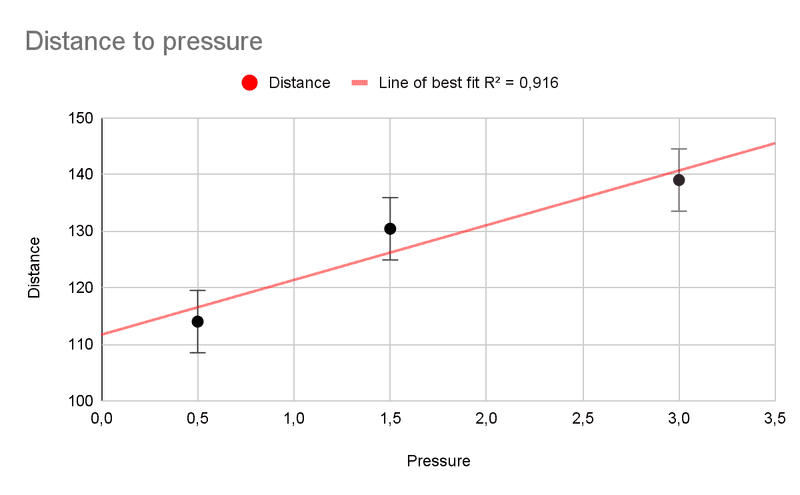Gold Member
Thank you.

You should probably collect a couple more data points (in between the three points), and maybe another just outside of the current domain if the tire permits. I would hesitate on proclaiming a linear correlation with 3 data points.

•tymi9
Homework Helper
Gold Member
The resistance to rolling in this case is formed more of the tire’s carcase deformation than of the contact patch resistance to rolling (two surfaces in contact, like in traditional friction discussion).

More kinetic energy is consumed by cyclic deformation and heating of the ruber-fabric structure and air contained within the tire (assuming similar conditions for tests).

The lower the pressure, the higher air compression happens due to irregularities of the pavement (compression-decompression cycles, like inside a reciprocant air compressor).

I would estimate rolling resistance in function of tire‘s pressure, rather than pure rolling friction.

•erobz
tymi9
And how to do that?

Mentor
I'm working with mountain bike's tires and asphalt.
How aggressive is the tread on your MTB tires? If it is pretty aggressive (lots of space between the knobs), it will have pretty high rolling resistance on pavement. Most of my MTB rides involve about 50% dirt and 50% asphalt with lots of hills, so I typically ride with street pressure (65psi) unless I'm going to be doing steep slippery hill climbs.

One of the best discoveries I made for MTB tires that you are riding both dirt/pavement with is to find tires that have a ridge structure down the middle, but still are aggressive enough off centerline to provide good traction in dirt and mud. This is the tire I'm currently running:

Bontrager Connection Trail Tire 26-inch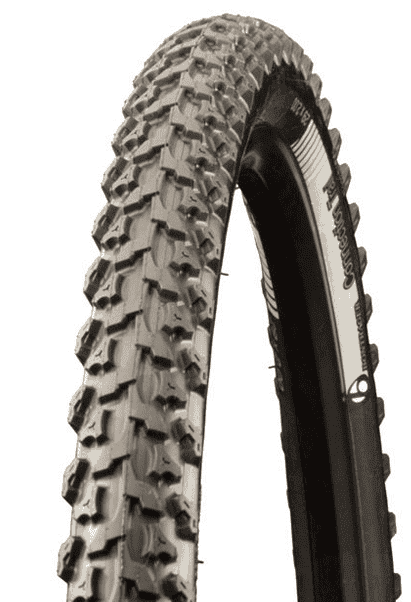https://www.summitbicycles.com/product/bontrager-connection-trail-tire-26-inch-369774-1.htm

•Lnewqban
Homework Helper
Gold Member
And how to do that?

The coeficient that you are looking for should be the slope of the experimental curve that you have plotted.

•tymi9
Gold Member
The coeficient that you are looking for should be the slope of the experimental curve that you have plotted.
Under the assumption of a ##C_{rr}## being independent of velocity acting as some constant force (different for each pressure), with a "static" frictional force from bearings etc...

$$- f_{rr} - f_b = m a = const. \implies f_{rr} = -ma - f_b \tag{1}$$

We should also have the data for ##a## under a constant force from the graph:

$$a = -\frac{v_o^2}{2x} \tag{2}$$

Sub (2) into (1):

$$f_{rr} = m \frac{v_o^2}{2x} - f_b \tag{3}$$

If we assume ##f_{rr} = mg C_{rr}## is a function of the tire pressure ##P## we get the following system:

\begin{align} mg C_{rr}(P_1) &= m \frac{v_o^2}{2x_1} - f_b \tag{4} \\ mg C_{rr}(P_2) &= m \frac{v_o^2}{2x_2} - f_b \tag{5} \end{align}

Subtracting (4) from (5):

$$g ( C_{rr}(P_2) - C_{rr}(P_1) ) = \frac{v_o^2}{2} \left( \frac{1}{x_2} - \frac{1}{x_1} \right) \tag{6}$$

If we assume that ##C_{rr}## is a linear decreasing function of ##P##

$$C_{rr}(P_2) = C_{rr}(P_1) - \beta( P_2 - P_1) \tag{7}$$

Sub (7) into (6):

$$-g\beta ( P_2 - P_1 ) = \frac{v_o^2}{2} \left( \frac{1}{x_2} - \frac{1}{x_1} \right) \tag{8}$$

It's not seeming to me like the slope of that graph in post#6 is ##C_{rr}(P)## which would be ##\frac{x_2 - x_1}{P_2 - P_1}##, Have I messed up?

Last edited:
•Lnewqban
Homework Helper
Gold Member
... It's not seeming to me like the slope of that graph in post#6 is ##C_{rr}(P)## which would be ##\frac{x_2 - x_1}{P_2 - P_1}##, Have I messed up?
It seems that my previous statement is incorrect, according to what the OP defines as Crr.
That slope is actually the proportion between conservation of energy per unit of distance (easiness to roll) and tire’s pressure.

Gold Member
Backing up a bit , to eq(6)
$$g ( C_{rr}(P_2) - C_{rr}(P_1) ) = \frac{v_o^2}{2} \left( \frac{1}{x_2} - \frac{1}{x_1} \right) \tag{6}$$

This can be written like:

$$\Delta C_{rr} = \frac{v_o^2}{2g} \left( \frac{1}{x_2} - \frac{1}{x_1} \right) \tag{6'}$$

If we assume from the graph given that the distance ##x## is linear in ##P## (arguably the simplest possibility)

Then we can write:

$$x(P) = C + \beta P$$

where ##\beta## is the slope of the graph in question

After some algebraic manipulation I find:

$$\frac{ \Delta C_{rr}}{ \Delta P} = -\frac{v_o^2}{2g} \left( \frac{\beta }{( C + \beta P_2)(C + \beta P_1) } \right) \tag{7}$$

This indicates that whatever the function is for ##C_{rr}## it seems to have some non-linear dependency on ##P## under this model (given that its "discrete first derivative" does - I'm winging it with this terminology - please call me out if it's too abused)?

Last edited:
Gold Member
I think we can just finish it out formally as:

$$\Delta C_{rr} = \frac{v_o^2}{2g} \left( \frac{1}{C+ \beta ( P + \Delta P)} - \frac{1}{C+ \beta P} \right)$$

Getting Common denominator:

$$\Delta C_{rr} = \frac{v_o^2}{2g} \left( \frac{(C+ \beta P)-(C+ \beta ( P + \Delta P) ) }{( C+ \beta ( P + \Delta P))(C+ \beta P)} \right)$$

Expanding the numerator and denominator:

$$\Delta C_{rr} = \frac{v_o^2}{2g} \left( \frac{ \cancel{C}+ \cancel{\beta P} \cancel{-C} \cancel{- \beta P} -\beta \Delta P }{( C+ \beta P)^2 + \beta \Delta P(C+ \beta P)} \right)$$

Divide through by ##\Delta P## and apply the limit

$$\frac{dC_{rr}}{dP} = \lim_{\Delta P \to 0} \frac{ \Delta C_{rr}}{ \Delta P} = -\frac{v_o^2}{2g} \left( \frac{ \beta }{( C+ \beta P)^2 + \cancel{ \Delta P(C+ \beta P)}^0} \right)$$

$$\implies \frac{dC_{rr}}{dP} = -\frac{v_o^2}{2g} \frac{ \beta }{( C+ \beta P)^2}$$

Last edited:
•tymi9
Gold Member
Yeah, something is off. The solution for ##C_{rr}## appears to be negative...Gold Member
Never mind, it does not appear to be negative... It think its ok

$$\implies \frac{dC_{rr}}{dP} = -\frac{v_o^2}{2g} \frac{ \beta }{( C+ \beta P)^2}$$
This implies

$$C_{rr}(P) = C_{rr}(P_o) -\frac{v_o^2}{2g} \left( \frac{1}{C+ \beta P_o} - \frac{1}{C+ \beta P}\right)$$

So we are left with some initial condition ## C_{rr}(P_o)## to determine...somehow...

Last edited:
tymi9
for any1 wondering I got the Crr like this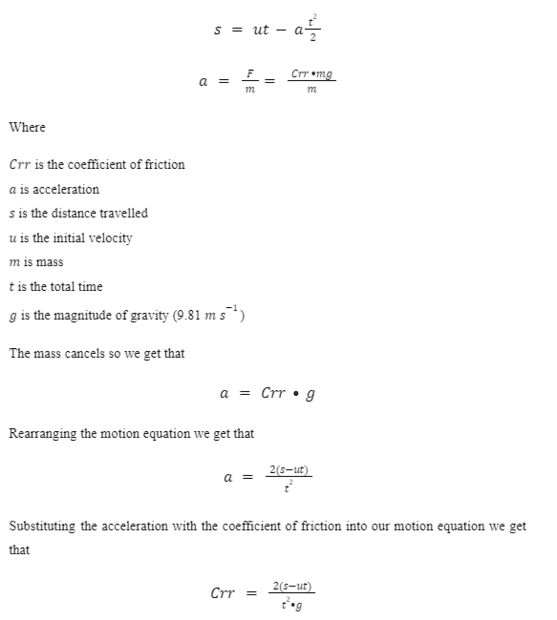Gold Member
for any1 wondering I got the Crr like thisView attachment 321265

That is equivalent to:

$$f_{rr} = m \frac{v_o^2}{2x}$$

$$\implies C_{rr}= \frac{v_o^2}{2gx}$$

I thought the objective was to obtain ##C_{rr}## as a function of tire pressure though?

Since you are ignoring the bearing force (assuming they are negligible), you can just use the equation in post#17 ( the extra unknown ##f_b## is not present anymore -at least in the model)

##C## would just be the intercept of your graph in post #6 ( Units of Length)

##\beta## would be the slope of the graph in post #6 ( units of length/pressure )

Then the initial condition would just be

$$C_{rr}(0) = \frac{v_o^2}{2gC}$$

Finally it would all just reduce to:

$$C_{rr}(P) = \frac{v_o^2}{2g} \left( \frac{1}{C+\beta P} \right)$$

Last edited:
Gold Member
You might also like the empirical method from this post.

Dullard
The effective diameter of the wheel is also changing. That can show up in your result, and may not be a 'friction' effect (depending on your precise procedure).

Gold Member
Also, you might consider looking for an exponential (decay) fit, as opposed to the linear model.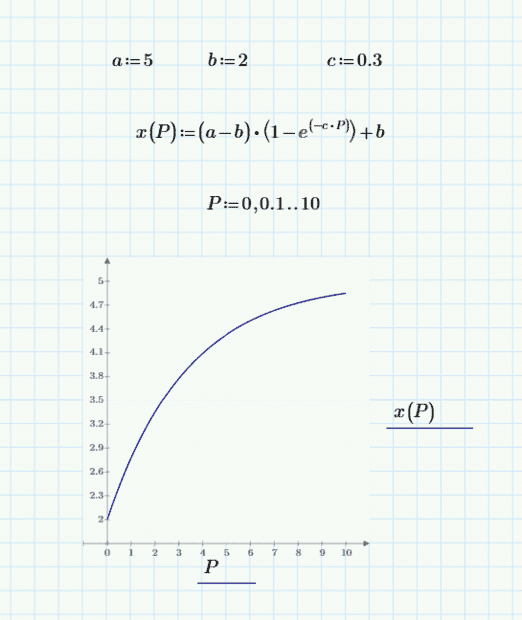I'm not sure, but I would expect the effect of tire pressure on ##C_{rr}## to decay with increasing pressure. i.e. as the pressures get larger, the change in stop distance should get smaller.

The linear model might be fine for any reasonable tire pressure, but you should take some more measurements to verify it's the best fit for the tire...IMO.

Last edited:
Gold Member
I just eyeballed this, it looks like a decent fit for the three points you have.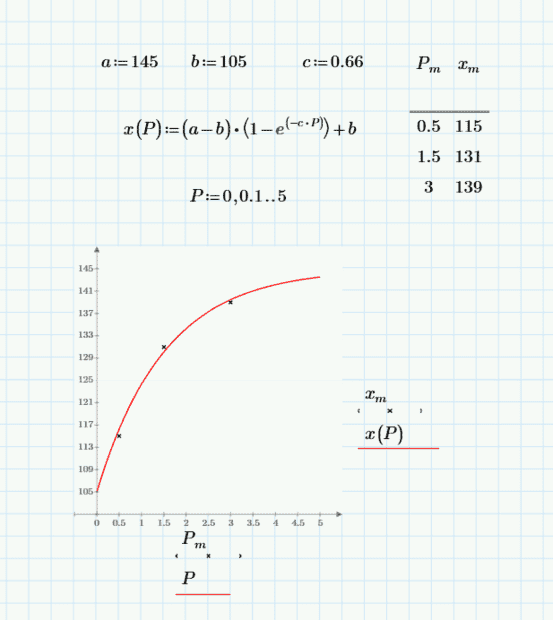You need more data to verify, and whatever program you are using to plot will optimize the parameters for you.

tymi9
Yeah, I figured that logarithmic function fits better. It gave me an R^2 of 0.998. Thanks for the help everyone

•erobz